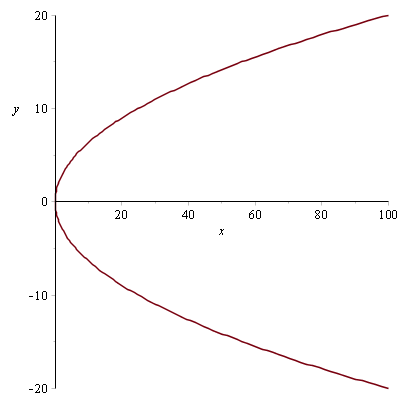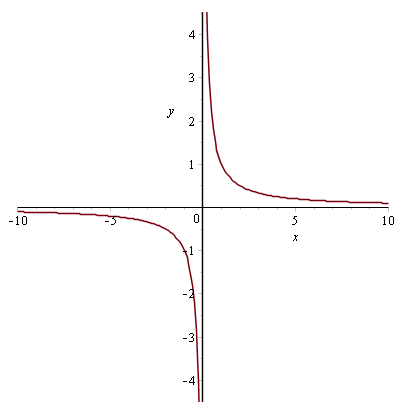# Coordinate systems

## Introduction

In FP1 you are introduced to the parabola and rectangular hyperbola, two types of curves that have interesting properties.

You will learn the general properties of these curves, how to express them in both cartesian and parametric form, and how to calculate tangents and normal lines to them using simple differentiation.

### Parametric coordinates

You will be quite familiar with cartesian $x-y$ coordinates by now. We extend this with the notion of parametric coordinates.

Parametric coordinates allow you to make each coordinate a function of a parameter which is usually called $t$.

$(x,y)$ becomes

$$(x(t),y(t))$$

This means that both the $x$ and $y$ coordinates of a graph are determined entirely by the parameter $t$.

For the curves that we are about to define, parametric coordinates allow a distinct definition from an equation using simple cartesian coordinates.

### Tangents and normals

You will know from C1 and C2 that the equation for a straight line that passes through the point $(x_{1},y_{1})$ is

$$y-y_{1}=m(x-x_{1})$$

where $m$ is the gradient of the line.

We can use this formula to find the tangent and normal to a curve at any given point.

The tangent to some curve $f$ at the point $(x_{1},y_{1})$ is a straight line that just touches the curve at that point, with the same gradient as that point on the curve. We simply sub the gradient at that point $f'(x_{1})$ into the above equation for $m$

$$y-y_{1} = f'(x_{1})(x-x_{1})$$

The normal to some curve $f$ at the point $(x_{1},y_{1})$ is a straight line that goes through the curve at that point at a 90 degree angle. Instead of $m$ we use $-\frac{1}{m}$, where $m=f'(x_{1})$

$$y-y_{1} = -\frac{1}{f'(x_{1})}(x-x_{1})$$

## Parabola

In FP1 we look at the "sideways" parabola, which we define with the equation

$$y^{2} = 4ax$$

And in parametric form

$$(x=at^{2},y=2at)$$

Where $a$ is a constant real number. It looks like thisThe parabola has a focus point at $(a,0)$ and a directrix line $x=-a$ relating back to the constant in the definition.

These definitions are significant, because a straight line drawn from the focus to any point $p$ on the parabola is always the same length as a horizontal line drawn from the directrix to $p$ on the parabola.

The point $p$ on the parabola has cartesian coordinates $(p_{x},p_{y})$. Using pythagoras we equate the distance from the focus to $p$ with the horizontal length from $p$ to the directrix $x=-a$ \begin{align} \sqrt{(p_{x}-a)^{2} +p_{y}^{2}} &= p_{x}-(-a) \\ \sqrt{(p_{x}-a)^{2} +p_{y}^{2}} &= p_{x}+a \end{align} But the parametric coordinates for any point on the parabola are $(at^{2},2at)$, so sub these in place of $(p_{x},p_{y})$ \begin{align} \sqrt{(at^{2}-a)^{2} +(2at)^{2}} &= at^{2}+a \\ (at^{2}-a)^{2} +4a^{2}t^{2} &=(at^{2}+a)^{2} \\ 4a^{2}t^{2} &=(at^{2}+a)^{2}-(at^{2}-a)^{2} \end{align} The right side is now the difference of two squares \begin{align} 4a^{2}t^{2} &= ((at^{2}+a)+(at^{2}-a))((at^{2}+a)-(at^{2}-a)) \\ &= (at^{2}+a+at^{2}-a)(at^{2}+a-at^{2}+a) \\ &= (2at^{2})(2a) \\ &= 4a^{2}t^{2} ~ \blacksquare \end{align}

### Finding tangents and normals

A very common theme of question in the FP1 exam is asking you to find the tangent or normal line to a point on a parabola.

This usually involves finding the derivative of the parabola at some given point, and using the straight line formula defined above to form an equation for the type of line they ask for.

Finding the derivative of a parabola is quite simple. Using the cartesian form

\begin{align} y^{2} &= 4ax \\ y &= 2\sqrt{ax} \\ \therefore \frac{dy}{dx} &= \sqrt{\frac{a}{x}} \end{align}

You would then sub in your value of $x_{1}$ to find the gradient $m$ of the parabola at that point. You can then use that right away for a tangent line, or invert it and multiply by $-1$ to use it to find a normal line.

Alternatively, you may also find the derivative in the parameterised form

\begin{align} x(t) &= at^{2} \\ y(t) &= 2at \end{align}

Differentiating both with respect to $t$ yields

\begin{align} \dot{x}(t) &= 2at \\ \dot{y}(t) &= 2a \end{align}

Using the chain rule

$$\frac{dy}{dx} = \frac{dy}{dt}\div \frac{dx}{dt} = \frac{2a}{2at} = \frac{1}{t}$$

Indeed subbing $x=at^{2}$ into the cartesian derivative we found above

$$\frac{dy}{dx} = \sqrt{\frac{a}{at^{2}}} = \frac{1}{t}$$

### Example

Q) Find the equations of the tangent and normal at the point $x=5$ to the curve $y^{2}=20x$.

A) The curve is $y^{2}=20x$ so we can deduce that it is a parabola with $a=5$.

The $y$-value at $x=5$ is

\begin{align} y^{2}&=20\times 5 \\ \Rightarrow y &= \sqrt{100} \\ &= 10 \end{align}

The derivative of the curve at $x=5$ is

$$\frac{dy}{dx} = \sqrt{\frac{5}{x}} = \sqrt{\frac{5}{5}} = 1$$

So we have $m=1$, $x=5$, and $y=10$. Subbing these into the straight line formula we can find the equation for the tangent at $x=5$

\begin{align} y-10 &= 1\times (x-5) \\ y &= x+5 \end{align}

Now to find the equation for the normal at $x=5$, we use $-\frac{1}{m}$ instead of $m$

\begin{align} y-10 &= -\frac{1}{1} (x-5) \\ y &= 15-x \end{align}

### Example

Q) Find the equations of the tangent and normal to the parabola with coordinates $(t^{2},2t)$ at the point $t=3$.

A) A general point on the parabola has coordinates $(t^{2},2t)$ so we can deduce that $a=1$.

We have $t=3$, so using the parametric derivative of a parabola defined above we find that

$$\frac{dy}{dx} = \frac{1}{t} = \frac{1}{3}$$

Subbing in $t=3$ to find the values of $x$ and $y$

\begin{align} x(t) &= 3^{2} = 9 \\ y(t) &= 2\times 3 = 6 \end{align}

So we have $m=\frac{1}{3}$, $x=9$, and $y=6$. Subbing these into the straight line formula we can find the equation for the tangent at $t=3$

\begin{align} y-6 &= \frac{1}{3} (x-9) \\ y &= \frac{1}{3}x+3 \end{align}

Now to find the equation for the normal at $t=3$, we use $-\frac{1}{m}$ instead of $m$

\begin{align} y-6 &= -3(x-9) \\ y &= 33-3x \end{align}

### Example

Q) Show that the equation of every tangent line to the parabola with coordinates $(2t^{2},4t)$ takes the form $t(y-2t)=x$.

A) Subbing the general coordinates $(2t^{2},4t)$ and $m=\frac{dy}{dx} = \frac{1}{t}$ into the straight line formula

\begin{align} y-4t &= \frac{1}{t}(x-2t^{2}) \\ yt - 4t^{2} &= x-2t^{2} \\ yt-2t^{2} &= x \\t(y-2t)&=x\end{align}

## Hyperbola

The rectangular hyperbola is the second curve we look at in FP1, defined by the equation

$$xy=c^{2}$$

And in parametric form by the coordinates

$$\left(ct,\frac{c}{t}\right)$$

Where $c$ is a constant real number. It looks like this### Finding tangents and normals

In FP1 you need to know how to find tangents and normals to some point $(x_{1},y_{1})$ on the rectangular hyperbola. Once again you will need to find the derivative at the point and use the straight line formula.

Using the cartesian form

\begin{align} xy &= c^{2} \\ y &= \frac{c^{2}}{x} \\ \therefore \frac{dy}{dx} &= -\left(\frac{c}{x}\right)^{2} \end{align}

Then sub in the value of $x_{1}$ to find the gradient $m$ of the hyperbola at that point. You can then use that right away for a tangent line, or invert it and multiply by $-1$ to use it to find a normal line.

To find the derivative in parameterised form

\begin{align} x(t) &= ct \\ y(t) &= \frac{c}{t} \end{align}

Differentiating both with respect to $t$ yields

\begin{align} \dot{x}(t) &= c \\ \dot{y}(t) &= -\frac{c}{t^{2}} \end{align}

Using the chain rule

$$\frac{dy}{dx} = \frac{dy}{dt}\div \frac{dx}{dt} = -\frac{c}{t^{2}} \div c = -\frac{1}{t^{2}}$$

Indeed subbing $x=ct$ into the cartesian derivative we found above

$$\frac{dy}{dx} = -\left(\frac{c}{ct}\right)^{2} = -\frac{1}{t^{2}}$$

### Example

Q) Find the equations of the tangent and normal to the curve $xy=9$ at the point $x=1$.

A) We deduce that this is a hyperbola with $c=3$.

The value of $y$ at $x=1$ is therefore

$$y = \frac{9}{1} = 9$$

And the derivative at $x=1$ is

$$\frac{dy}{dx} = -\left(\frac{3}{1}\right)^{2} = -9$$

So we have $m=-9$, $x=1$, and $y=9$. Subbing these into the straight line formula

\begin{align} y-9 &= -9(x-1) \\ y &= 18-9x \end{align}

For the normal we use $-\frac{1}{m}$ instead of $m$

\begin{align} y-9 &= \frac{1}{9}(x-1) \\ y &= \frac{1}{9}x+\frac{80}{9} \end{align}

### Example

Q) Find the equations of the tangent and normal to the hyperbola with coordinates $(2t,\frac{2}{t})$ at the point $t=5$.

A) We deduce that $c=2$, and find that $m=\frac{dy}{dx} = -\frac{1}{25}$.

From $t=5$ we find that $x=10$ and $y=\frac{2}{5}$, and with $m=-\frac{1}{25}$ we can form the tangent line

\begin{align} y-\frac{2}{5} &= -\frac{1}{25}(x-10) \\ y &= \frac{4}{5}-\frac{1}{25}x \end{align}

To form the normal line we use $-\frac{1}{m}$ instead of $m$

\begin{align} y-\frac{2}{5} &= 25(x-10) \\ y &= 25x-249.6 \end{align}

### Example

Q) Show that the equation of every normal line to the hyperbola with coordinates $(4t,\frac{4}{t})$ takes the form $t(y-xt^{2})=4(1-t^{4})$.

A) See that $-\frac{1}{m} = t^{2}$. Subbing the coordinates $(4t,\frac{4}{t})$ into the straight line formula

\begin{align} y-\frac{4}{t} &= t^{2}(x-4t) \\ yt-4 &= xt^{3}-4t^{4} \\ t(y-xt^{2}) &= 4(1-t^{4}) \end{align}